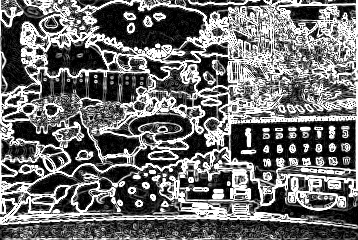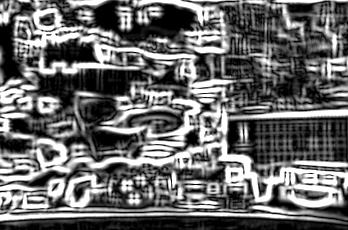Institute for Telecommunication Sciences / Research Topics / Video Quality Research / Guides and Tutorials / Spatial Information (SI) Filter

# Spatial Information (SI) Filter

NTIA/ITS developed a spatial information (SI) filter that detects long edges. This filter is similar to the classical Sobel filter in that separate horizontal and vertical filters are applied, then the total edge energy is computed as the Euclidean distance. The filter is referred to by size, for example SI13 uses (13 x 13) bandpass filters.

The SI filter code also estimates the edge angle. SI is separated into HV and HVbar, such that HV contains the horizontal-vertical edges (and zero otherwise), and HVbar contains the diagonal edges. Low energy edges are omitted. This edge angle estimation is robust in that the large filter size reduces the impact of noise and pixel location quantization on the angle measurement.

The SI filter assumes that subjects focus on long edges and tend to ignore short edges. As the filter size increases (e.g., SI5, SI7, SI9), individual pixels and small details have a decreasing impact on the edge strength and angle calculation. By contrast, Sobel (3 × 3) responds identically to short and long edges. To see the impact compare Figures 1, 2 and 3 (below). Notice the fine texture within the sheep and at the bottom of the image are prominent with the Sobel filter (Figure 2) but subdued with the SI13 filter (Figure 3). The line between the top page and bottom page of the calendar is obvious in the SI13 image but does not stand out in the Sobel image. The SI13 filter identifies edges that are approximately 13 pixels long. Each image is (360 x 243).

MATLAB code for the SI filter is available here. This code may be used for any purpose, commercial or non-commercial. Please contact Margaret Pinson if you find any bugs or errors in this code.Figure 1. Calendar and Mobile with analog noise added, Luma plane (Y)Figure 2.   Calendar and Mobile, Sobel filter (3 x 3)Figure 3. Calendar and Mobile, SI13 filter (13 x 13)